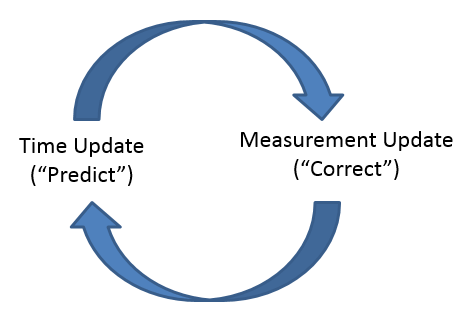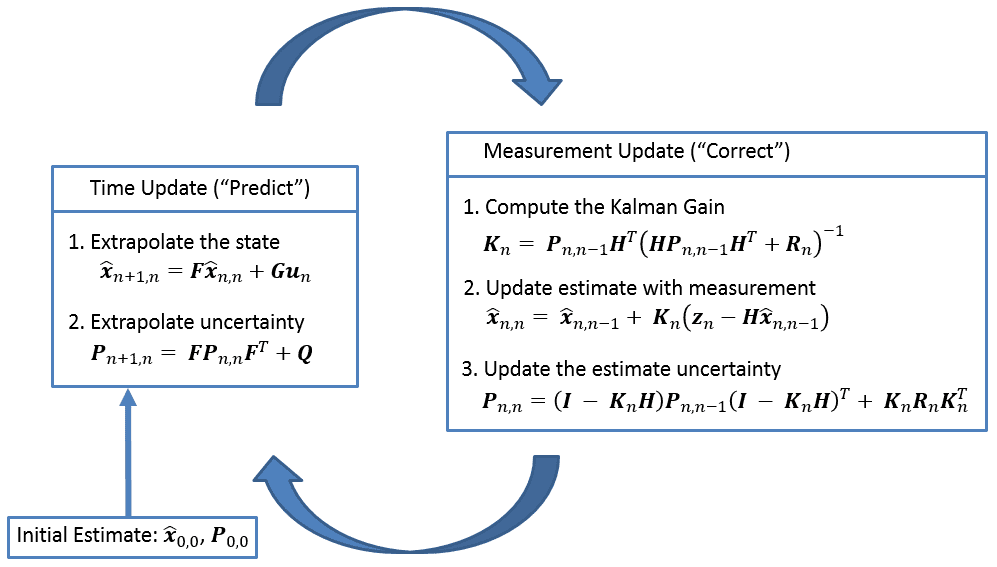﻿ Summary

# Summary

We have derived all five Kalman Filter equations in matrix notation. Let us put them all together in a single page.

The Kalman Filter operates in a “predict – correct” loop, as shown in the diagram below.Once initialized, the Kalman Filter will predict the system state at the next time step. It also provides the uncertainty of the prediction.

Once the measurement is received, the Kalman Filter updates (or corrects) the prediction and the uncertainty of the current state. As well, the Kalman Filter predicts the next states, and so on.

The following diagram provides a complete picture of the Kalman Filter operation.The following table describes all Kalman Filter Equations.

Equation Equation Name Alternative names
Predict $$\boldsymbol{\hat{x}_{n+1,n}=F\hat{x}_{n,n}+Gu_{n}}$$ State Extrapolation Predictor Equation
Transition Equation
Prediction Equation
Dynamic Model
State Space Model
$$\boldsymbol{P_{n+1,n} = FP_{n,n}F^{T} + Q}$$ Covariance Extrapolation Predictor Covariance Equation
Update
(correction)
$$\boldsymbol{\hat{x}_{n,n} = \hat{x}_{n,n-1} + K_{n} ( z_{n} - H \hat{x}_{n,n-1} )}$$ State Update Filtering Equation
$$\boldsymbol{ P_{n,n} = \left( I - K_{n}H \right) P_{n,n-1} \left( I - K_{n}H \right)^{T} + K_{n}R_{n}K_{n}^{T} }$$ Covariance Update Corrector Equation
$$\boldsymbol{ K_{n} = P_{n,n-1}H^{T}\left( HP_{n,n-1}H^{T} + R_{n} \right)^{-1} }$$ Kalman Gain Weight Equation
Auxiliary $$\boldsymbol{z_{n} = Hx_{n}}$$ Measurement Equation
$$\boldsymbol{R_{n}} = E\left( \boldsymbol{v_{n}v_{n}^{T}} \right)$$ Measurement Uncertainty Measurement Error
$$\boldsymbol{Q_{n}} = E\left( \boldsymbol{w_{n}w_{n}^{T}} \right)$$ Process Noise Uncertainty Process Noise Error
$$\boldsymbol{P_{n,n}} = E\left( \boldsymbol{e_{n}e_{n}^{T}} \right) = E\left( \left( \boldsymbol{x_{n} - \hat{x}_{n,n}} \right) \left( \boldsymbol{x_{n} - \hat{x}_{n,n}} \right)^{T} \right)$$ Estimation Uncertainty Estimation Error

The next table summarizes notation (including differences found in the literature) and dimension.

Term Name Alternative term Dimensions
$$\boldsymbol{x}$$ State Vector $$n_{x} \times 1$$
$$\boldsymbol{z}$$ Output Vector $$\boldsymbol{y}$$ $$n_{z} \times 1$$
$$\boldsymbol{F}$$ State Transition Matrix $$\boldsymbol{\Phi,A}$$ $$n_{x} \times n_{x}$$
$$\boldsymbol{u}$$ Input Variable $$n_{u} \times 1$$
$$\boldsymbol{G}$$ Control Matrix $$\boldsymbol{B}$$ $$n_{x} \times n_{u}$$
$$\boldsymbol{P}$$ Estimate Uncertainty $$\boldsymbol{\Sigma}$$ $$n_{x} \times n_{x}$$
$$\boldsymbol{Q}$$ Process Noise Uncertainty $$n_{x} \times n_{x}$$
$$\boldsymbol{R}$$ Measurement Uncertainty $$n_{z} \times n_{z}$$
$$\boldsymbol{w}$$ Process Noise Vector $$\boldsymbol{y}$$ $$n_{x} \times 1$$
$$\boldsymbol{v}$$ Measurement Noise Vector $$n_{z} \times 1$$
$$\boldsymbol{H}$$ Observation Matrix $$\boldsymbol{C}$$ $$n_{z} \times n_{x}$$
$$\boldsymbol{K}$$ Kalman Gain $$n_{x} \times n_{z}$$
$$\boldsymbol{n}$$ Discrete Time Index $$\boldsymbol{k}$$

Dimensions notation:

• $$n_{x}$$ is a number of states in a state vector
• $$n_{z}$$ is a number of measured states
• $$n_{u}$$ is a number of elements of input variable